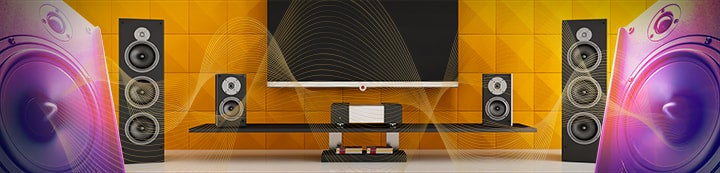1 - 2 of 2 Posts

brucek

·
Registered
Joined
·
7,514 Posts
The problem is that every graphics equalizer may calculate bandwidth differently.

As an example, some EQ's (such as the DSP1124P) define bandwidth as:

Bandwidth (Hz) = centre frequency*(BW/60)*sqrt(2)

So, the Q formula becomes: Q = 60/[(BW/60)*sqrt(2)].

For the R-DES eq, the bandwidth is defined as (1.766*centre frequency/Q).

Many are just the standard Bandwidth = centre frequency/Q.

So for each equalizer you would need to know how bandwidth is defined in relation to the standard Q calculation (√2/BW). One equalizer may define its 2/3 octave as the total width of the filter at its -3dB endpoints, (so a 2/3 octave filter would formulate to Q of 2.12). Another may define only the positive half gain endpoint, so a 2/3 octave filter actually covers 4/3 octave overall. There are many different definitions of bandwidth.

brucek

brucek

·
Registered
Joined
·
7,514 Posts
REW could 'calibrate' itself
REW allows the selection of various EQ's, so that the filters comply with that specific equalizer.

brucek

1 - 2 of 2 Posts